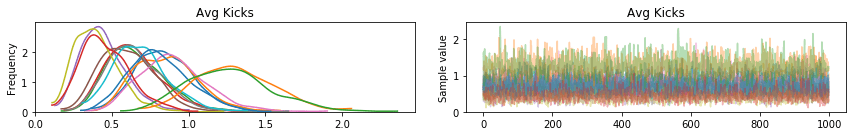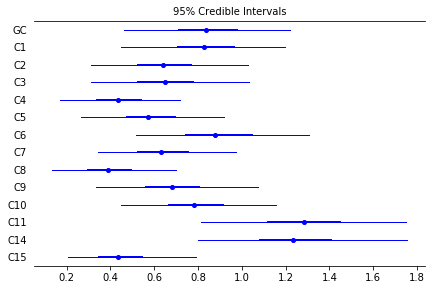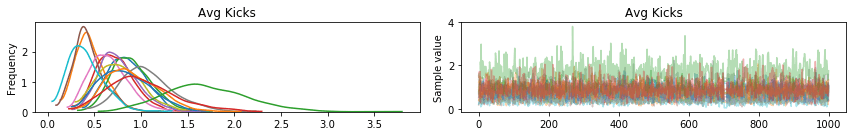Bayesian Poisson A/B Testing in PYMC3 on PythonThis post is a direct response to the request made by @Zecca_Lehn on twitter (Yes I will write tutorials on your suggestions). What he wanted to know was how to do a Bayesian Poisson A/B tests. So for those of you that don’t know what that is let’s review the poisson distribution first.

The poisson distribution is useful for modeling count data, particularly over a period of time. Say the number of times someone in the Prussian Calvary gets kicked in the head by a horse and dies as a result over a certain period of time, let’s say a year. In fact, this is a very classic data set that can be modeled by the poisson distribution quite well. So why don’t we go ahead and use this data to see how we can test whether one corps of the Prussian Calvary was better at not getting kicked by horses.

I don’t want you to think that I have gone off the rails with this example. So before we proceed, let’s take a step back and talk about why this data will work. This data has some interesting features, first it is count data, over several time periods, over several groups (corps in this case). Generally, A/B testing is most commonly used in the internet marketing space these days, so let’s look at how the Prussian Horse Kick data compares to internet marketing data. In internet marketing data we have the number of views clicks, etc. which has been collected for a number of pages (typically 2, hence A/B testing), over a time period like a month. So our data actually might look like it could have been generated by a similar process to the horse kick data. So let’s dive in deep to what that process might look like.

The Poisson Distribution

I don’t want to get overly “mathy” in this section, since most of this is already coded and packaged in pymc3 and other statistical libraries for python as well. So here is the formula for the Poisson distribution:$P=\dfrac{\lambda^{k}}{k!}e^{-\lambda}$

Basically, this formula models the probability of seeing$k$ counts, given$\lambda$ expected count. In other words,$\lambda$ is the mean of the number of counts for the page, corps, or whatever it is that you are looking at. This distribution is useful so long as three things are true:

1. What happens in one time period is independent of what happens in any other time period
2. The probability of an event (a click, pageview, horse kick, etc.) is the same for every time period
3. Events do not happen simultaneously

If you violate any of these three assumptions, you will need to mess around with the basic model that I am going to provide. Like adding in an autocorrelation feature, or some other modeling non-sense that you need to be careful about. It isn’t difficult to do it, but you do need to know that something is going on in order to know how to address it. If you need some help with your particular application feel reach out at ryan@barnesanalytics.com or call (801) 815-2922 to get some consulting for your particular application. I’m more than happy to help out.

That’s Enough Theory, Let’s Clean the Data

Okay so the first step that we’ll need to do is to do some minor cleaning of this dataset so that it will be in a format that our model will be able to digest. So download it from the link above and we’ll load it into python and get started.

import pandas as pd
import pymc3 as pm
import matplotlib.pyplot as plt
import numpy as np



So for the model’s sake we need to stack this dataset. So that the data has a unit of measurement of corps-year. We’ll start by moving the year variable to the index and then dropping it from the variable from the dataframe, as the extra year variable floating around will mess things up.

df.index = df['Year']
df.drop(['Year'],1,inplace=True)


Our next step would be to “stack” the data. Right now the data is in a pivot-table like format, what we want to do is unpivot this table. The command to do that in python is “stack”. This will collect all of the information except for the counts in the index, which I also don’t like, so I’m going to chain that last command with the reset index command, which will move my variables out of the index. This one-two combo is really powerful when you need to unpivot things in python. So it is worth keeping this combo in the back of your head, for future use. I know that I have it memorized. I also rename my columns from the defaults that python gives to things, just to keep things nice. Here’s how to do that:

df=df.stack().reset_index()
df.columns = ['Year', 'Corps', 'Count']
df.dropna(inplace=True)


At this point you can inspect your data with df.head(), and your data should look something like this:

     Year   Corps  Count
0    1875      GC      0
1    1875      C1      0
2    1875      C2      0
3    1875      C3      0
4    1875      C4      0


This is what we need the data to look like in order to do a Bayesian Poisson A/B Test. There is one last bit of data munging that needs to happen. We need to add a numerical index for the Corps. This numerical index is important, because PYMC3 will need to use it, and it can’t use the categorical variable. All of this code just builds this numerical index, I think it is quite clear what is going on in this code.

corps = df['Corps'].unique()
corps = pd.DataFrame(corps, columns=['Corps'])
corps['i'] = corps.index
df = pd.merge(df, corps, on=['Corps'], how='left')


So now our data is cleaned up and ready to use. It should be pretty painless to write a model down and run it.

Let’s Build Our Poisson A/B Model

Okay, as a brief side note, another reason why I chose this dataset to do this analysis with is because of the number of Corps. There are way more than 2 of them. This is the really exciting thing about doing this in a Bayesian Framework, we can build a hierarchical model, and test multiple versions concurrently. This means that we’re not only limited to an A/B test, like we would be in a frequentist setting, but we can do A/B/C/D tests! So let’s write down the model, and I’ll explain what is going on:

with pm.Model() as model:
mu=pm.Flat('Avg Kicks', shape=len(corps))
obs=pm.Poisson('Observed', mu=mu[corps_index], observed=df['Count'])
trace = pm.sample(1000, tune=1000)


So the first thing that we do is declare that we’re building a PYMC3 model. We give the model a number of parameters to work with, in fact one for each corps. These parameters are given an uninformative prior, so that we aren’t biasing them in anyway. These parameters are the average of a poisson distribution. So here’s where we make an assumption, we assume that each of our counts comes from a Poisson distribution specific to the corps from which the observation was taken. And then we run MCMC over the whole thing.

This procedure ran in under 30 seconds on my old laptop. So if you run it on a newer machine, or gpu, it should crank through it really super fast. There isn’t a lot of data, or parameters for this model to chew on, so it is no wonder that it runs pretty quick. We can examine the results through some of the default plots in pymc3.

pm.traceplot(trace)
plt.show()
pm.forestplot(trace, ylabels=corps.Corps.values)
plt.show()


This code will result in the following two figures:Clearly, our posteriors show that some of the corps are clearly better at not getting kicked by horses. Notably is looks like C11 and C14 could learn something from C4 and C15. C11 and C14 are our worst offenders, but they are also the most variable in terms of how often they get kicked. We can actually compute the probability that any corps gets more kicks than another, say C11 gets more than C4.

pred = [1 if obj1>obj2 else 0 for obj1,obj2 in zip(trace['Avg Kicks'][:,corps[corps['Corps'] == 'C11']['i']],trace['Avg Kicks'][:,corps[corps['Corps'] == 'C4']['i']])]
print(np.mean(pred))


When I do this, I get 100% of the sampled points in the posterior distribution for corps C11 are higher than for C4. This means that corps C4 unambiguously and systematically suffers fewer horse kicks than group C11.

We can also check whether or not C14 gets more horse kicks systematically than C11. This looks like it might be a fairer test. Let’s try this out:

pred = [1 if obj1>obj2 else 0 for obj1,obj2 in zip(trace['Avg Kicks'][:,corps[corps['Corps'] == 'C14']['i']],trace['Avg Kicks'][:,corps[corps['Corps'] == 'C11']['i']])]
print(np.mean(pred))


When I did that, there was only a 44% chance that C14 gets more kicks than C11. That means that there probably isn’t a very strong difference between these two groups.

A note on unbalanced data

So @Zecca_Lehn also wanted to know about how these bayesian testing would do on unbalanced data. At first glance, I had no idea what he meant. I mean I did because I run into this problem all the time as I have been working on a credit card fraud detection model recently. But in the context of a Poisson Count model, an unbalanced dataset doesn’t make a ton of sense. Counts are counts. You have none, or you have some.

But as I was thinking about the problem, it dawned on me that you could start observing data at different times. As such, I fudged the data a little bit. You can find my modified version of the horse kick data here. What I did is, I deleted some data so that we start observing the different corps on different years. This creates unbalanced data in the sense that I have unequal data for each of the corps. I think this is the situation @Zecca_Lehn was asking about.

The nice thing is that we don’t need to modify the script that we have just written except to drop the missing observations from the dataset. If we do that we’ll be good to go, and we can just run with the same code. So I snuck it into the code above in anticipation of running it on this modified dataset. So all that I did was modify the line that loads the data to use the new csv file.

These are the resultant plots from the script. If you compare them to the plots that you obtain from the full dataset you will notice that they look similar, however, I did delete some data so the numbers do change slightly. Also, the 95% credible intervals for the parameters have grown larger, and the more data that I deleted, the wider those intervals got.So the way to think of it is that the dataset doesn’t have as much information so we are less confident in the conclusions that we can draw from the dataset. In this sense, we can actually say something about how performant this model is in the face of an unbalanced data. In essence, it will give you its best “guess” as to what the parameter values should be, but it will be less confident in the “guesses” it supplies as the data for a certain class goes down.

Also we can still perform the probability analysis that we did before. Since I just ran the same script on the modified data we can actually see how the predictions changed in light of this unbalanced data. We still see that there is virtually a 100% chance that C11 is greater than C4, but due to the wider confidence intervals, and shifting of the data due to dropping some observations there would only be about a 9.7% chance that C14’s population mean is greater than C11’s population mean.

Here’s the full code:

#!/usr/bin/env python3
# -*- coding: utf-8 -*-
"""
Created on Thu Jul 20 07:02:05 2017

@author: ryan
"""

import pandas as pd
import pymc3 as pm
import matplotlib.pyplot as plt
import numpy as np

df.index = df['Year']
df.drop(['Year'], 1, inplace=True)

df=df.stack().reset_index()
df.columns = ['Year', 'Corps', 'Count']
df.dropna(inplace=True)

corps = df['Corps'].unique()
corps = pd.DataFrame(corps, columns=['Corps'])
corps['i'] = corps.index
df = pd.merge(df, corps, on=['Corps'], how='left')

corps_index = df.i.values

with pm.Model() as model:
mu=pm.Flat('Avg Kicks', shape=len(corps))
obs=pm.Poisson('Observed', mu=mu[corps_index], observed=df['Count'])
trace = pm.sample(1000, tune=1000)

pm.traceplot(trace)
plt.show()
pm.forestplot(trace, ylabels=corps.Corps.values)
plt.show()

pred = [1 if obj1>obj2 else 0 for obj1,obj2 in zip(trace['Avg Kicks'][:,corps[corps['Corps'] == 'C11']['i']],trace['Avg Kicks'][:,corps[corps['Corps'] == 'C4']['i']])]
print(np.mean(pred))
#%%
pred = [1 if obj1>obj2 else 0 for obj1,obj2 in zip(trace['Avg Kicks'][:,corps[corps['Corps'] == 'C14']['i']],trace['Avg Kicks'][:,corps[corps['Corps'] == 'C11']['i']])]
print(np.mean(pred))